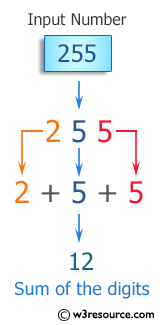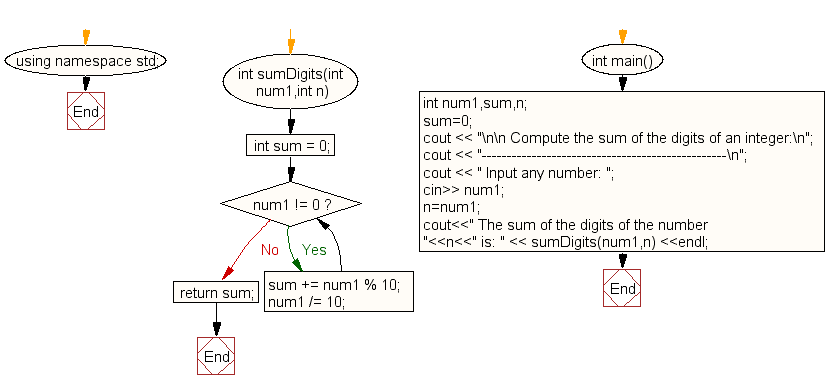﻿ C++ : Compute the sum of the digits of an integer# C++ Exercises: Compute the sum of the digits of an integer using function

## C++ For Loop: Exercise-84 with Solution

Write a program in C++ to compute the sum of the digits of an integer using function.

Pictorial Presentation:Sample Solution:-

C++ Code :

``````#include <iostream>
using namespace std;

int sumDigits(int num1,int n)
{
int sum = 0;
while (num1 != 0)
{
sum += num1 % 10;
num1 /= 10;
}
return sum;
}

int main()
{
int num1,sum,n;
sum=0;
cout << "\n\n Compute the sum of the digits of an integer:\n";
cout << "-------------------------------------------------\n";
cout << " Input any number: ";
cin>> num1;
n=num1;
cout<<" The sum of the digits of the number "<<n<<" is: " << sumDigits(num1,n) <<endl;
}
``````

Sample Output:

```Compute the sum of the digits of an integer:
-------------------------------------------------
Input any number: 255
The sum of the digits of the number 255 is: 12
```

Flowchart:C++ Code Editor:

Contribute your code and comments through Disqus.

What is the difficulty level of this exercise?

﻿